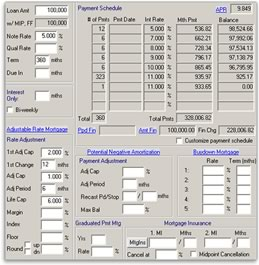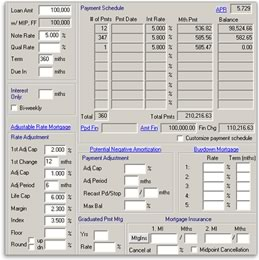# 2822 Entering Adjustable Rate Mortgage (ARM) loans in Point

An Adjustable Rate Mortgage Loan, (ARM), is a type of mortgage in which the note rate increases or decreases through the loan schedule or until the life cap is reached.

The life cap is the maximum note rate this loan can reach.  The note rate varies depending on the margin and index rates at the time.  The margin and index change often; therefore, no one can determine the exact note rate six months from now. When entering the Life Cap into Point, enter the difference between the actual Life Cap and the Note Rate. (For Example: Life Cap - Note Rate = the rate that you enter into Point as the Life Cap.)

Point can only show two scenarios, the worst case scenario and the best case scenario.  Most users choose to show the worst case scenario to the Borrower.

Worst Case Scenario:

When disclosing worst case scenario on an ARM, the APR will also calculate a worst case scenario showing an increase in the APR.

The worst case scenario applies when we do not know what the margin and index rates will reach in the future. In this example, the note rate increases 2% after the first 12 months, then increases 1% every six months after that, until the life cap is reached.

• 1st Adj Cap  Indicates the maximum increase or decrease of the Note Rate, as it changes the first time. (For example: the Note Rate, in this example, can increase 2% over the note rate.)
• 1st Change – Indicates the number of months that the loan will remain fixed at the initial Note Rate. (For example, the note rate increases 2% after the first 12 months.)
• Adj Cap – Indicates the maximum percentage that the rate increases for each sub-sequent adjustment period, after the first.  (For example, the note rate adjusts 1% after the first adjustment cap.)
• Adj Period – Indicates the frequency that the note rate will increase per the Adj Cap. (For example, the note rate will increase 1% every 6 months.)#### Best Case Scenario: When disclosing best case scenario on an ARM, the APR will also calculate a best case scenario showing an decrease in the APR.

To view a best case scenario, enter a Margin and Index.  The best case occurs when we know the current Margin and Index.

This scenario is based on the assumption that the margin and index will remain basically the same from six months to a year from now.

• Notice the Note Rate increases to 5.8 after the first 12 months.
• The 1st ChangeAdj CapAdj Period, and Life Cap fields are ignored because the best case scenario is Margin + Index = Note Rate. (In this example, the Margin is 2.3 and the Index is 3.5.)
• In this example, the Margin + Index = 5.8.  Thus, the note rate after the first change of 12 months is 5.8.